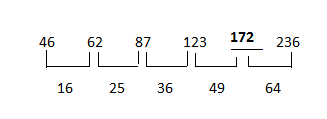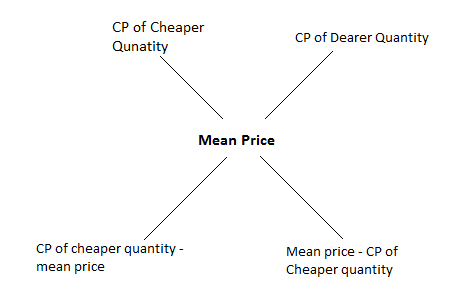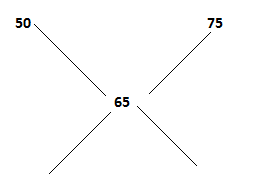# Maths Tricks & Shortcut For Competitive Exams

Maths tricks and shortcuts are the easiest and fastest ways in which you can solve mathematical problems in the upcoming Government exams.

The quantitative aptitude or the numerical ability section is most commonly a part of all major Government exams, and if a candidate can get shortcut tricks to solve this section quickly, he may be able to score a higher overall percentage in the examination.

 Candidates who are looking for questions from the quantitative aptitude section for the various Government exams 2021 can refer to the set of links mentioned below:

In this article, we have given the 10 Maths tricks that will make the quantitative aptitude section less stressful and easier to solve. Aspirants who are preparing for the upcoming competitive exams can refer to these tricks.

## 10 Simple Maths Tricks & Shortcuts

### 1. Square Root

Finding the square root of a number by estimating and multiplying can be a long procedure. Given below is a simpler method to find the square root of a number:

Example:Find the square root of 2116

To find the square root of 2116:

Step 1: See the digit in one’s place. In this case, it is 6. Now, check between 1-9, the square of what all numbers have “6” at one’s place. The answer is 42 = 16 and 62 = 36.

Step 2: Now, check the square of which number between 1 to 9 is closest to the first two digits of the given number. In this case, the sum of the number between 1 to 9 is closest to 21. The answer is 42 = 16 and 52 = 25.

So, one number among 44, 46, 54 and 56 is the square root of 2116.

Step 3: For the two numbers you got in step 2, multiply each of them with the next number in the number series. That is, 4×5 = 20 and 5×6 = 30. Since 20 is a closer number to 21, the answer has to be either 46 or 44. Multiply and check your answer.

Check yourself with the below-mentioned example:

Example: What is the square root of 1024?

Solution:

Step 1: 22 = 4 and 82 = 64

Step 2: 32 = 9

Step 3: 3×4 = 12. Since 12 is greater than 10, the square root will be 32.

### 2. Cube Root

Follow the steps given below to find out the cube root of a number quickly.

Example: What is the cube root of 9261?

Step 1: Find the numbers between 1 to 9 whose cube is equal to the digit present at the one’s place; here, it is 1. So, we get 1×1×1 = 1.

Step 2: See the first digit of the number, in this case, 9. 9 lies between the cube of 2 (2×2×2 = 8) and (3×3×3 = 27). Since 8 is closest to 9, the cube root of 9261 is 21.

Note: To find the cube root of 5 digit number, use the first two digits instead of the first digit in step 2

Try one example by yourself to understand the trick even better:

Example: What is the cube root of 32768?

Step 1: 23 = 8

Step 2: 33 = 27 and 43 = 64

Since 27 is closer to 32, the cube root of 32768 will be 32.

Candidates who are looking for shortcut tricks to calculate the square & cube of a number can visit the linked article.

Given below are two examples of quadratic equations solved with easy tricks to find the answer quickly:

Example: x² – 18x + 45 = 0

Step 1: Multiply the coefficient of x² and the constant in the equation. In this case, 1×45 = 45

Step 2: Multiply “-1” with the coefficient of x. In this case, -1× (-18) = 18

Step 3: Hence, the value of x will be 15 and 3 (3+15=18 & 3×15=45). Remember, for signs, if the answer obtained in both step 1 & 2 is positive, then both values of x will be positive. If even one is negative, then values of x will be negative.

Here, the value obtained in step 1 & 2 is positive hence the value of x will be positive. So, the answer is x = 15, 3

Example: x²-5x-6 = 0

Step 1: Multiply the coefficient of x² and the constant in the equation. In this case, 1×(-6) = (-6)

Step 2: Multiply “-1” with the coefficient of x. In this case, (-1)× (-5) = 5

Step 3: Hence, the value of x will be 6 and 1 (6-1=5 & 6×1=6). Remember, for signs, if the answer obtained in both step 1 & 2 is positive, then both values of x will be positive. If even one is negative, then one of the values of x will be negative.

Step 4: Here the answer in step 1 is negative. Thus, one value of x will be negative. If the answer in step 1 is negative, the smaller value of x will be negative. If the answer in step 2 is negative, the larger value will be negative.

So, x= 6, -1

### 4. Number Series

If a candidate is confused about the system followed in a number series, the easiest way to find the difference between two numbers in the series.

Example: 46 62 87 123 ? 236

Solution:Step 2: Once you find the difference, you will notice that the pattern with the square of numbers has been followed.

42 = 16

52 = 25

62 = 36

72 = 49

82 = 64

For any other question asked in number series format and the candidate faces any kind of confusion in following the pattern, they can directly find the difference between two numbers in the series, it shall make solving it easier.

Candidates can visit the Number Series page to know more about the concept and the types of questions which may be asked under this topic.

### 5. Compound Interest

Given below are a few formulas that may save you some time during the exam while solving the compound interest problems:

(a) If compound interest is x% for 1st interval of time and is y% for the second interval of time, Then,

Net Effective Rate of Interest after the 2 intervals = x + y + (xy/100)

Note: This is applicable if both the time intervals are equal)

(b) If a sum of money, say P, amounts to A1 in a certain duration of time, say T, at Compound Interest and the same sum of money amounts to A2 in “2T” time at Compound Interest,

Then,

P/A1 = A1/A2

(c) If a sum of money, say P, amounts to A1 in a certain time duration, say T, at compound interest and the same sum of money amounts to A2 after T+1 years at compound interest

Then,

Rate of Interest = {(A2-A1) / A1} × 100

For example: Raj pays compound interest at 16% per annum to Shyam, which is compounded quarterly. What is the effective rate of interest per annum paid by Raj?

Solution:

Annual interest rate = 16%

So, the interest is paid quarterly, which makes a 4 time installment. Therefore, the rate of interest per quarter = 16/4 = 4%

Using (a) x + y + (xy/100).

4 + 4 + {(4×4)/100} = 8 + 0.16 = 8.16% for two quarters

For four quarters, 8.16% + 8.16% = 16.32%

### 6. Simple Interest

Take reference from the formulas given below and save some time while solving the questions in the final exam for the quantitative section:

(a) Difference between simple and compound interest for 2 years = {(PR)2/ (100)2}

(b) Difference between simple and compound interest for 3 years = {PR2 (300+R) / 1003}

For example: The difference between simple interest and compound interest for two years, on a certain sum of money at 4% per annum is Rs.800, when compounded annually. What is the sum of money on which the interest has been gained?

Solution:

Following (a) CI-SI = {(PR)2/ (100)2}

⇒800 = {(P×4)2/ (100)2}

⇒P = Rs. 707.11

Aspirants can check the Quantitative aptitude syllabus for various Government exams at the linked article.

### 7. Time & Work

Given below is a simpler way to find out the time taken to complete a piece of work done by three people, when working together:

Example: Three labourers, Ajit, Sumit & Ramesh take 10, 8 and 20 days respectively to complete the same piece of work. How long will it take for all three of them if they work together?

Solution:

LCM of 10, 8 and 20 = 40

Efficiency of Ajit = 40/10 = 4

Efficiency of Sumit = 40/8 = 5

Efficiency of Ramesh = 40/20 = 2

Time Taken by all three together = {(LCM) / (Efficiency of all three)} = 40/11 days

So to calculate the time taken to complete the same work by 3 people = (Total Unit of Work) / Efficiency of all the works)

For further information regarding the concept of Time and Work and the top tips to solve questions based on this topic, candidates can visit the linked article.

### 8. Approximation

Simple multiplication is something that consumes maximum of our time while solving maths questions in competitive exams. Given below is a shortcut to multiply two numbers which may help you in questions related to approximation and simplification.

Example: Solve 32 × 34

Step 1: Multiply the first number (in this case, 32) with the digit at ten’s place in second number (in this case, 3)

We get, 32×3 = 96

Step 2: Add a “0” to the answer obtained in step 1. So the number now becomes “960”

Step 3: Multiply 32 with the one’s digit in the second number, we get, 32×4 = 128

Step 4: Add the result obtained in step 2 & step 3.

So answer is 960 + 128 =  1088

Solve more same questions based on Simplification and Approximation at the linked article.

### 9. Rule of 72

The rule of 72 is used to solve questions where a given sum of money needs to be doubled in a specific period of time with a certain rate of interest.

 Formulas to remember: Number of years invested = 72/ Annual Investment Rate Investment Rate = 72/ Number of years Invested Investment rate x Number of years invested = 72

For Example: If Raj invested Rs.500/- in a friend’s business, then how much time will it take to double Raj’s investment, if the rate of interest is 8%?

Solution:

So, according to rule of 72,

Time duration in which the amount will be doubled at 8% interest rate = 72/8 = 9 years

### 10. Mixture & Alligation

Given below is maths trick to solve the mixture and alligation questions frequently asked in Government exams in a quicker and easier manner.

 Formula to Remember:For example: A shopkeeper mixes two varieties of pulses worth Rs.50 per kg and Rs.75 per kg, respectively. In what ratio must the shopkeeper mix these two pulses, making the average cost of mixture Rs. 65 per kg.

Solution:Cheaper Quantity/ Dearer Quantity = (75-65) / (65-50) = 10/15 = 2:3

Those who are not much familiar with the concept of Mixture and Alligation can visit the linked article to know more about the concept, types of questions which may be asked and get some sample questions along with their solutions for assistance.

### Tips To Ace Quantitative Aptitude Section

The maths tricks and shortcuts given above will only be useful if a candidate has built strong basics. For aspirants who do not have a strong command over the quantitative questions, they can check the quantitative aptitude tips given below for their assistance:

• Build Your Basics Strong – If the foundation is not stable, moving further can be difficult. Thus, a candidate must spend sufficient time on each topic and ensure that the basics are clear as only then would candidates be able to analyse as to which maths tricks to apply where
• Get the Formulas Right – Do not just learn the formulas but also understand where they can be applied so that time can be saved while solving the questions from the different topics
• Understand the Question – Before starting to solve a question, read it and understand it and then start solving it keeping in mind the easiest and shortest approach to ensure no time is wasted
• Practise and Practise – Once a candidate understands the concept, he/she must solve more and more questions based on the same to get a hang of the concept
• Create Table & Charts – In case the question is lengthly and solving it is taking a lot of time, create table and charts to simplify the given data
• Proper Time Management – Many candidates end up wasting time on a particular question. Unknowingly a candidate ends up wasting precious time which could be entrusted for other questions.

The quantitative aptitude tips given above will help candidates prepare themselves for the various upcoming competitive exams and help them score more in this section in particular.

Candidates may refer to the above-mentioned maths tricks and quantitative aptitude tips as they will simplify the questions for them and also save them a lot of time. At first, these tricks may seem to be longer but with practise, candidates will be able to solve more questions in a shorter period of time.

For any other update or information regarding Government exams, candidates can turn to BYJU’S for help.